Subject:
Geometry
Material Type:
Lesson Plan
Level:
Middle School
7
Provider:
Pearson
Tags:
Language:
English
Media Formats:
Interactive, Text/HTML

# Exploring Polygons# Lesson Overview

Students explore different polygons by drawing them, measuring angles, and manipulating interactive sketches to find the angle sum for any polygon. Students also explore the angle measures in regular polygons.

# Key Concepts

• The angle sum in a triangle is 180°. A quadrilateral can be composed of two triangles, so the angle sum of a quadrilateral is 360°.
• The number of triangles that compose a polygon is two less than the number of sides (angles). The sum of the interior angles in a polygon with n sides is 180° (n – 2).

# Goals and Learning Objectives

• Find angle sums in polygons.
• Generalize to find the angle sum for any polygon.
• Find interior angle measures for regular polygons.

# Lesson Guide

Show students the opening figure and discuss the question, “What do you know about the sum of the angles in this figure (or figures)?”

ELL: Make sure that your pace is appropriate when posing these questions, especially when interacting with ELLs. Allow ELLs time to process their observations, and discuss with a partner.

# Mathematics

There are several possible interpretations that can enrich the discussion. Students may see the following:

• The sum of the angles in the quadrilateral is 360°.
• The sum of the angles in each triangle is 180°.

Ask students to think about how the angle sums might be connected.

# Angle Sums

• What do you know about the sum of the angles in this figure (or figures)?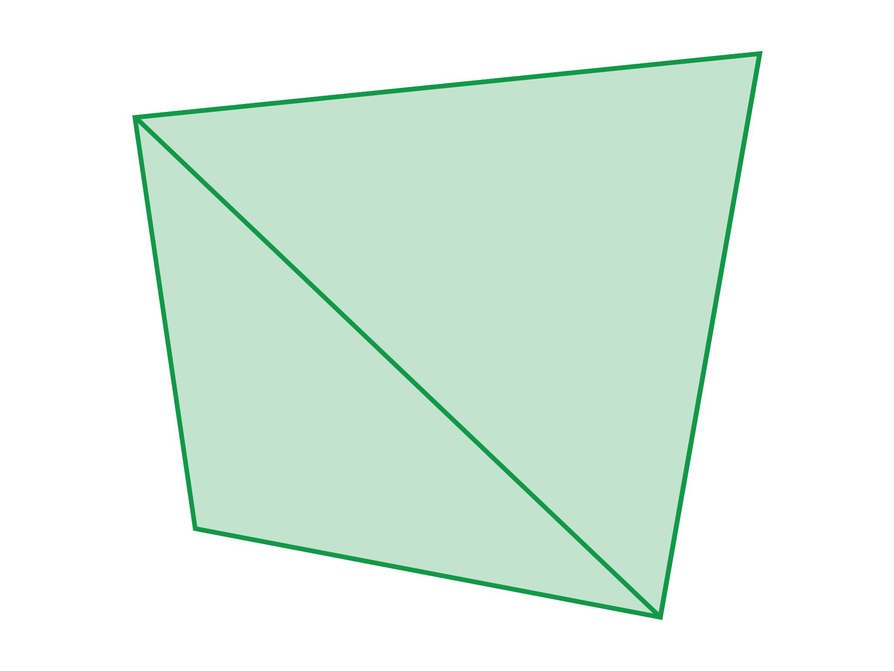# Lesson Guide

Discuss the Math Mission. Students will determine how to find the sum of the angle measures for any polygon.

## Opening

Determine how to find the sum of the angle measures for any polygon.

# Lesson Guide

SWD: Students with disabilities may struggle to independently find patterns for the interior angle sum of polygons. Create the chart with them to show the interior angle sum for each polygon. Then ask guiding questions to see if they recognize the pattern (every time you add a side, the interior angle sum increases by 180°). Use your understanding of your students' needs to inform any pre-teaching and/or interventions you may provide.

# Interventions

Student does not measure the angles correctly.

• Estimate the size of the angle. How large do you think it is?
• Is it greater than or less than 90°?
• Is the protractor lined up on a side of the polygon?

Student has a solution.

• How would you find the angle sum for a polygon with n sides?

• If students are starting to see the pattern, they may predict the pentagon is 540° or 180° more than the quadrilateral sum.They may then extend this pattern to correctly predict the hexagon's angle sum.
• Pentagon: 540°
Hexagon: 720°
• Students should notice that the angle sum is increasing by 180° as the number of sides increases by 1. They might also see that the sums are multiples of 180°.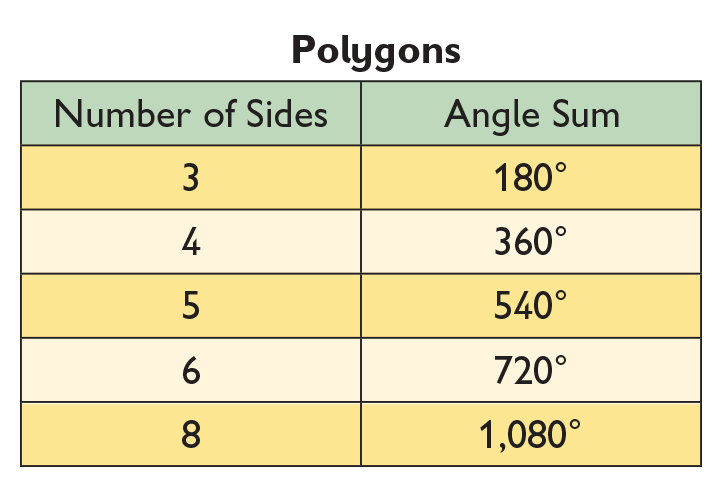# Make a Table of Angle Sums

On a blank sheet of paper, draw a pentagon and a hexagon using the ruler side of your protractor.

• What do you think the sum of the angles for each figure is?
• Measure each angle of the pentagon and hexagon. Find the angle sum for each figure.
• Complete the first four rows of the table based on what you know. Make a prediction for the last row. What do you notice? Do you see a pattern in the way that the angle sums increase?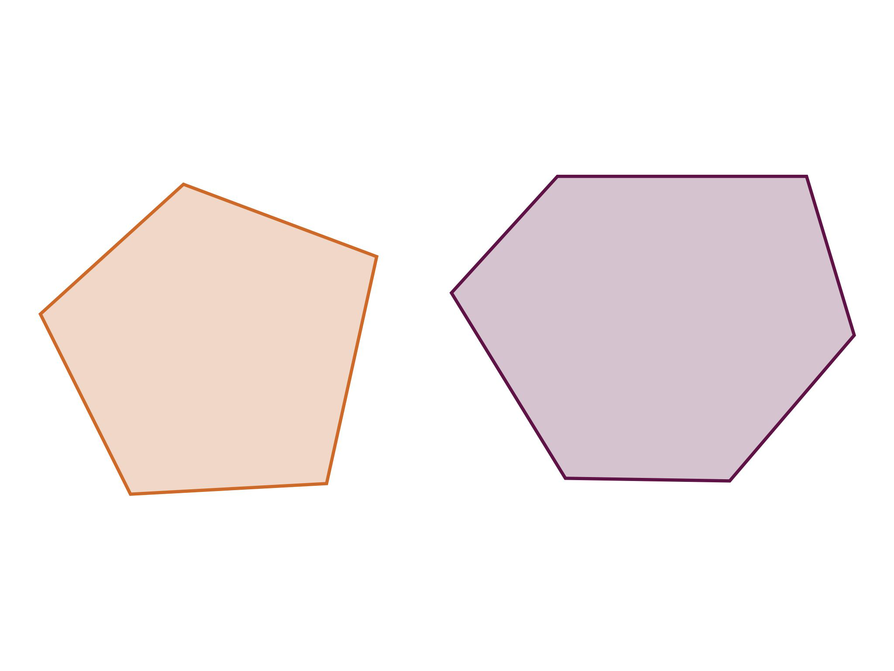INTERACTIVE: Angle Sum Table

# Lesson Guide

Allow time for students to explore the Polygon Angle Sum interactive.

# Interventions

[common error] Student does not think that a polygon and a regular polygon are the same, as far as the angle sum.

• In the Polygon Angle Sum interactive, make each angle the same in the polygons. What is the angle sum? Did it change?

• Students should explore a variety of possible configurations for each polygon.
• Students should predict that the angle sums for the pentagon, hexagon, and octagon are 540°, 720°, and 1080° respectively.
• No, the angle sum does not change as the figure is changed.
• A convex polygon may have, at most, three acute angles. A concave polygon may have more. All of the angles can be obtuse. The purpose of the question is to help students see that the angles are getting larger as the number of sides increases.

# Change Angles

• Explore the angles of the given polygons by dragging the vertices to change the angles.
• Predict what the sum of the angles will be as you change each polygon. Then select the Show Angle Sum buttons to check your prediction.
• Does the angle sum change as the figure is changed?
• How many acute angles can each figure have? How many obtuse angles can each figure have?

INTERACTIVE: Polygon Angle Sum

## Hint:

Compare the number of sides in the polygon to the angle sum. By how many degrees does the angle sum increase for each additional polygon side?

# Lesson Guide

As students work, look for students who are not making the connection between the polygons and dividing the polygons into triangles. Encourage these students to use the Hints.

# Interventions

Student does not see the connection between the triangles and the polygons.

• How many triangles are in a quadrilateral?
• What is the angle sum in a triangle? In two triangles?
• What is the angle sum for the pentagon?
• How many triangles can you form from a pentagon?

• The following image shows some possible solutions; students may also use quadrilaterals or draw triangles that all share a common vertex within the polygon and then subtract 360° from the angle sum of all the triangles formed: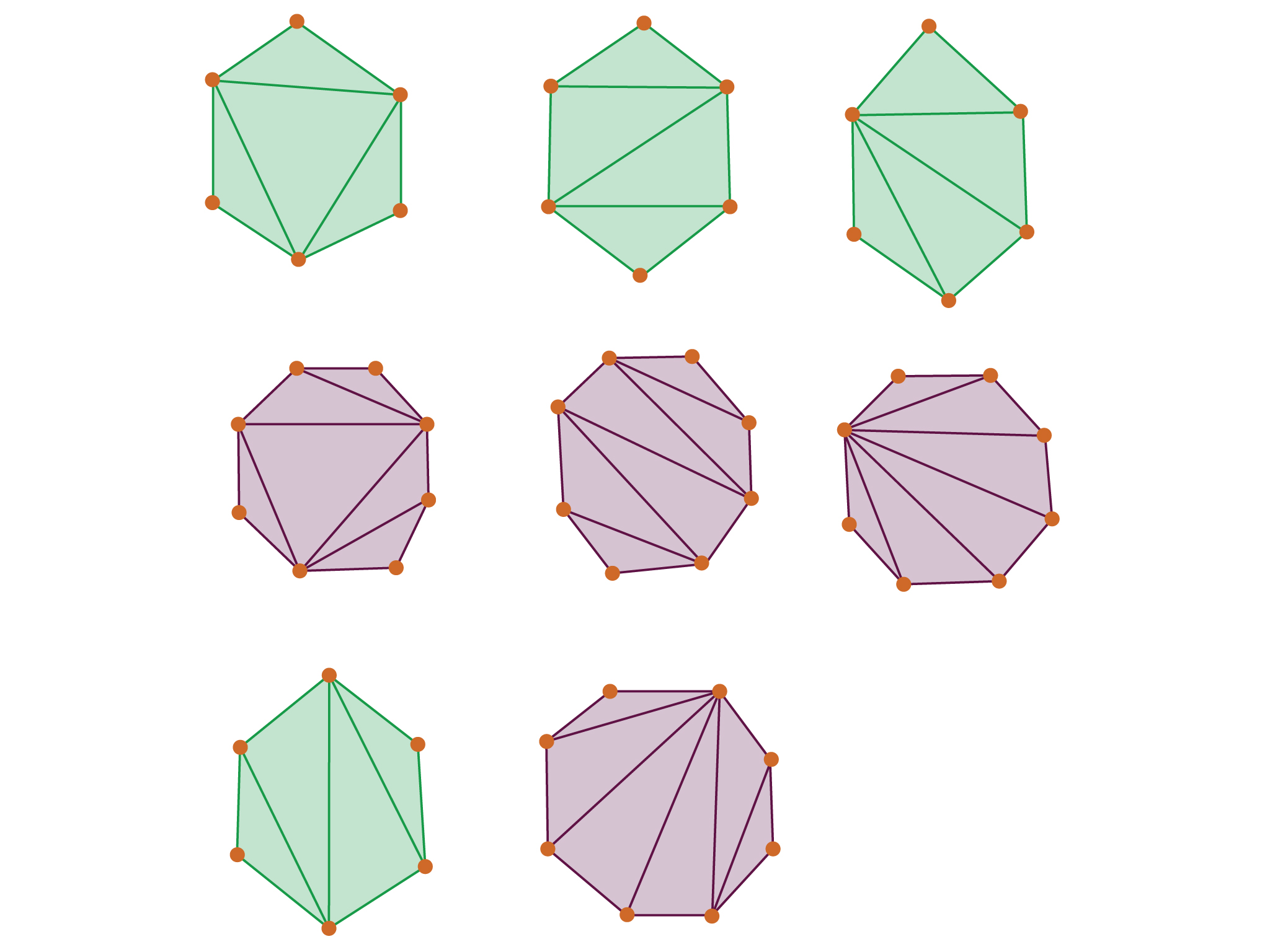# Explore Angle Sums

For each figure in the Polygon Angle Sum interactive, draw lines to help you determine the sum of the angles based on figures you know.

INTERACTIVE: Polygon Angle Sum

## Hint:

How many non-overlapping triangles can you divide each polygon into?

# Preparing for Ways of Thinking

Look for these types of responses to share during the Ways of Thinking discussion. Look for students who:

• See that the angle sums are multiples of 180°
• See that the multiple of 180° is 2 less than the number of sides in the polygon.
• Divide the polygons into triangles in different ways
• Can state a general “formula,” in different ways
• See the pattern of the angle size increasing in regular polygons as the number of sides increases
• Solve the Challenge Problem and see that the measure of each angle in a regular polygon approaches 180° as the figure gets closer to being a circle

# Mathematical Practices

Mathematical Practice 1: Make sense of problems and persevere in solving them.

• As students work, identify several students who use different strategies to make sense of the task of finding a general method to find the angle sum for a polygon.

Mathematical Practice 3: Construct viable arguments and critique the reasoning of others.

• Listen to students as they discuss finding the general rule and the arguments and evidence they use to state their case. Have these students share their strategies during the Ways of Thinking discussion.

Mathematical Practice 5: Use appropriate tools strategically.

• Students will be using tools strategically as they realize that the sketches are a more reliable method to use to analyze the polygons. They see the lack of possible precision in measuring the angles manually.

Mathematical Practice 6: Attend to precision.

• Look for students who see the importance of measuring the angles accurately. Students who do not attend to precision may not draw the proper conclusions (because angle measures will not add to multiples of 180°) and may not understand why. Similarly, students should see that even though the angle sums of the polygons and their neighbors are not exactly correct, they understand why and see that the sum should be a round number (for example, 540° vs. 537°).

Mathematical Practice 8: Look for and express regularity in repeated reasoning.

• Listen as students work for conversations in which students are:
• Discussing how to divide the polygons: “Look, you can break each of the polygons into triangles.”
• Discussing the angle sums: “I noticed that each angle sum is a multiple of 180°. Each time a side is added the angle sum increases by 180°.”
• Looking for shortcuts moving toward the general rule: “I see a shortcut. The number of triangles is always two less than the number of sides. Each triangle is 180°, so we can multiply.” Or, “The number of 180°s in each angle sum is two less than the number of sides.”

# Challenge Problem

• Angle sum: (100 − 2 ) ⋅ 180° = 98 ⋅ 180° = 17,640°
Angle measure: 17,640° ÷ 100 = 176.4°
If a regular polygon has 100 sides, the measure of each angle is 176.4°.

# Prepare a Presentation

Describe a method for finding the angle sum of any polygon. Support your method with examples from your work.

# Challenge Problem

If a regular polygon has 100 sides, what is the measure of each angle?

# Mathematics

Facilitate the discussion to help students understand the mathematics of the lesson. Lead a discussion and have students share their thinking. Ask questions such as the following:

• When you added the angle measures that you measured in the pentagon and hexagon that you drew, did they come out to exactly 540° and 720°? Why?
• Why does the angle sum increase by 180° as another side is added?
• Describe the method you used to find the angle sum in any polygon.
• If the number of sides in a regular polygon keeps increasing, what happens to the angle measure of each interior angle?
• If the number of sides in a regular polygon keeps increasing, what shape is the polygon getting close to?
• How is the mathematics represented differently by [students]? What did they have in common? What was different?
• How did [students] make sense of the problem? Can you state what [students] said in a different way?

ELL: When critiquing students whose proficiency in English is low, focus on what the student is trying to convey and not the grammar mistakes. If you are not sure you understand, ask the student to repeat in different ways. If other students in the class understand (and you don't), allow them to help you.

# Make Connections

• Take notes about your classmates' methods for finding the sum of the angles of any polygon.

## Hint:

• When you added the angle measures in the pentagon and hexagon that you drew, did the measures add up to exactly 540° and 720°? Explain.
• Why does the angle sum of a polygon increase by 180° each time a side is added?
• Can you explain how you determined your method for finding the angle sum in any polygon?
• If the number of sides in a regular polygon keeps increasing, what angle measure are the angles in the polygon getting close to? What shape is the polygon getting close to?

# Find Angle Sums

Students see that the number of triangles is always two less than the number of sides. They know each triangle has a sum of 180°, so they can multiply 180° by two less than the number of sides. Some students will see this pattern from the chart that they completed.

An alternate method involves dividing the polygon into as many triangles as there are sides. The key to this method is that students see that the 360° around the center are not part of the interior angles and need to be subtracted.

• The angle sum for a decagon is 1,440°.
(10 − 2) ⋅ 180° = 8 ⋅ 180° = 1,440°; or, 10 ⋅ 180° − 360° = 1,800° − 360° = 1,440°
• The angle sum for a dodecagon is 1,800°.
(12 − 2) ⋅ 180° = 10 ⋅ 180° = 1,800°; or, 12 ⋅ 180° − 360° = 2,160° − 360° = 1,800°

# Find Angle Sums

• What is the angle sum for a decagon (10 sides)?
• What is the angle sum for a dodecagon (12 sides)?

# Lesson Guide

Have students work on the Polygon Angle Measures table.

SWD: Struggling students may still need explicit instruction and guided scaffolding to recognize the relationships for finding the formula for the interior angle sum of any polygon. Check to make sure all students have a clear understanding, pull a small group of students to provide clarification as needed.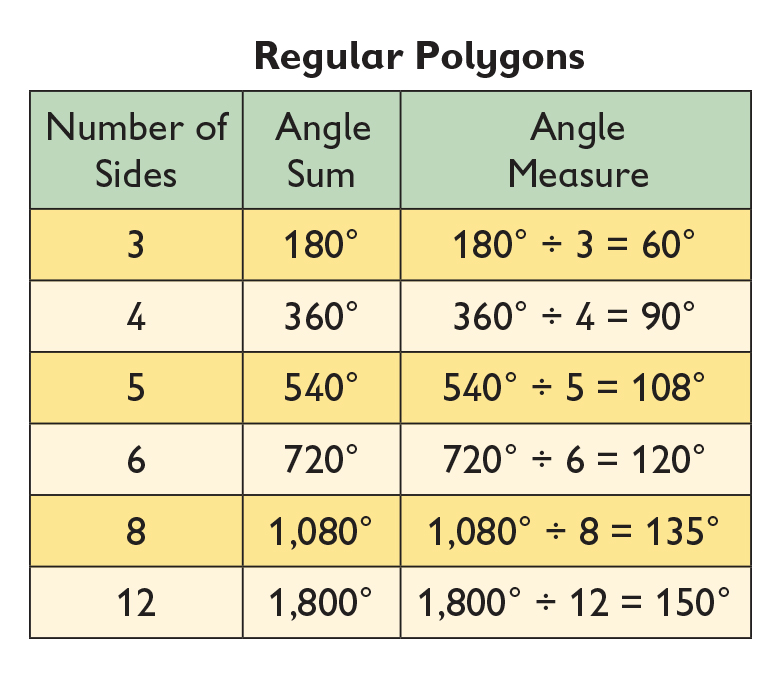The angle size is increasing as the number of sides increase.

# Find Angle Sums and Angle Measures

Investigate the angle measures for regular polygons. Remember that in a regular polygon, all sides are congruent and all angles are congruent.

• Complete the table. What do you notice?

INTERACTIVE: Polygon Angle Measures

# A Possible Summary

As the number of sides in a polygon increase, the angle sum increases as well. If the polygon is regular, the angle measures are increasing and becoming more obtuse. The angle sum increases by 180° for each added side in a polygon. Any polygon can be divided into two fewer triangles than there are sides. So, the angle sum can be found by multiplying 180° by the number of triangles because each triangle has a sum of 180°. As a regular polygon has more and more sides, it gets closer to being a circle and each angle gets closer to 180°.

# Summary of the Math: Polygon Angle Sums

• Write a summary about the sums of angles in polygons.

## Hint:

• Do you explain how to find the sum of the angles for any polygon?
• Do you explain how you can find the sum of the angles of a polygon by using the sum of the angles of a familiar figure?